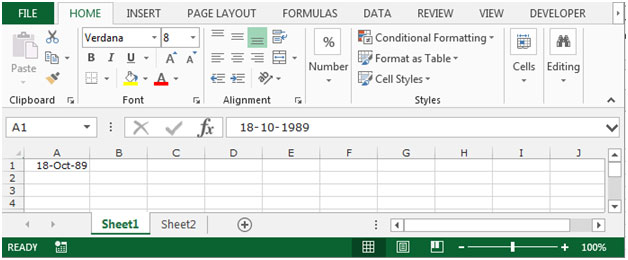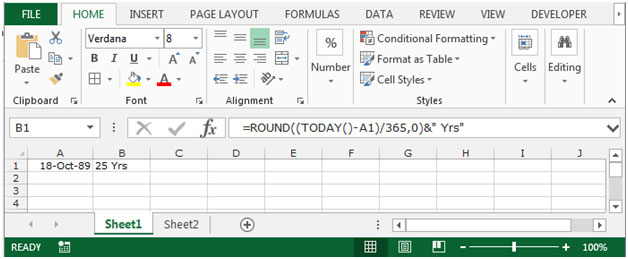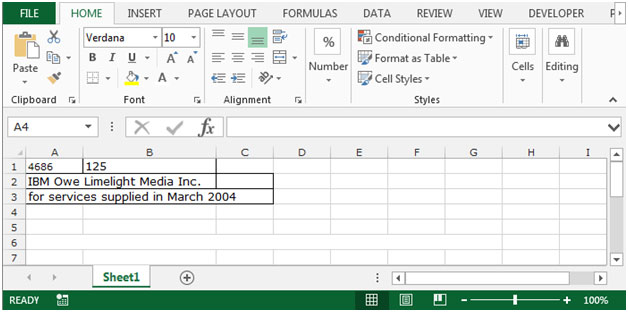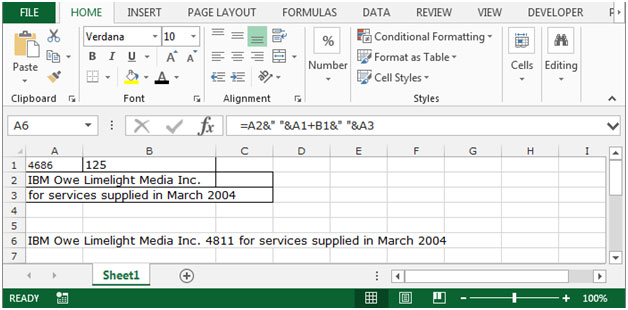# Combining Text and Formatted Values in Excel 2010

In this article we will learn about how to combine the text and formatted values in Microsoft Excel 2010.

Sometimes when we prepare analysis reports, we show the numbers with text and if we want to make any changes in the number that should be updated automatically in analysis points in Microsoft Excel 2010.

To do this we use the “&” symbol in our function. This symbol is used to add the text with calculated numbers.

Let’s take an example to understand that how we use the “&” symbol with calculated numbers.

We have a date of birth in cell A1, we want to calculate the age till today. We want the age to be automatically calculated on a daily basis. And also age should appear with the text years in cell B1.

To calculate the age we use the Today formula along with Round formula, in which today formula will work for today’s date and Round formula will round the figure which we got after the calculation of age.To do this follow below given steps:-

• Select the cell B1 and write the formula.
• =ROUND((TODAY()-A1)/365,0)&" Yrs", press enter.
• This function will return age in this “25 Yrs” format.Let’s understand another example, how to combine text and formatted values in a cell.

We have text and formulated value in the range A1:C3. In which A1 is containing the value 4686 and cell B1 is containing the value 125.If you want to combine the text and value with the total value of cell A1 and B1, follow below mentioned steps:-

• Select the cell A5, and write the formula.
• Equals to select the A2 type the sign of “&” space in between inverted commas, again type the sign of “&”, select the cell A1+B1 again the sign of “&” give the space in between inverted commas type sign off & and select the cell A3.
• =A2&" "&A1+B1&" "&A3
• Press Enter.Note: If you will change the value in cell A1 and B1, then value will get changed automatically.

This is all about the how to combine the text with formatted values in Microsoft Excel 2010 and 2013.

## Users are saying about us...

1.I hv 50 sheets in one file which has to be merged in one sheet, is there any formula to copy all sheets in one sheet?

2."Hi Sudhir,

I doubt there will be a simple answer to this one.

Please can you give some samples so that we have a better idea of what you want to achieve.

Thanks,

Alan"

3."i hv data in sheet1 from A1 to A3000 as mentioned below :
""Client Name"" ""Brand Name"" ""Bill No."" ""Bill Date""""Bill Amt.""

There are 50 to 60 sheets where columns are same but the data are different, now i hv to merge all sheets in one column, is there any formula? I hv tried ""consolidate"" formula in data menu but the problem is, it will only takes the total & other but i can't copy all text in one sheet? Could u pls help me?
Thanx & Regards, "

4."Hi Sudhir,

Sounds like a one off thing that you will never need to replicate?

If so, to be honest, I suggest you do it manually.

With 50 to 60 sheets, I cannot imagine it will take more than an hour or so, and you could easily spend that long trying to write a macro or formulae that would do it for you.

Is that practical?

Alan."

5.I hv 50 sheets in one file which has to be merged in one sheet, is there any formula to copy all sheets in one sheet?

6."Hi Sudhir,

I doubt there will be a simple answer to this one.

Please can you give some samples so that we have a better idea of what you want to achieve.

Thanks,

Alan"

7."i hv data in sheet1 from A1 to A3000 as mentioned below :
""Client Name"" ""Brand Name"" ""Bill No."" ""Bill Date""""Bill Amt.""

There are 50 to 60 sheets where columns are same but the data are different, now i hv to merge all sheets in one column, is there any formula? I hv tried ""consolidate"" formula in data menu but the problem is, it will only takes the total & other but i can't copy all text in one sheet? Could u pls help me?
Thanx & Regards, "

8."Hi Sudhir,

Sounds like a one off thing that you will never need to replicate?

If so, to be honest, I suggest you do it manually.

With 50 to 60 sheets, I cannot imagine it will take more than an hour or so, and you could easily spend that long trying to write a macro or formulae that would do it for you.

Is that practical?

Alan."

Terms and Conditions of use

The applications/code on this site are distributed as is and without warranties or liability. In no event shall the owner of the copyrights, or the authors of the applications/code be liable for any loss of profit, any problems or any damage resulting from the use or evaluation of the applications/code.